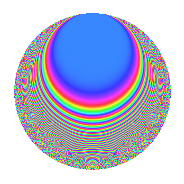Properties

 Label 4015.2.a.fLevel 4015 Weight 2 Character orbit 4015.a Self dual Yes Analytic conductor 32.060 Analytic rank 1 Dimension 31 CM No

Related objects

Newspace parameters

 Level: $$N$$ = $$4015 = 5 \cdot 11 \cdot 73$$ Weight: $$k$$ = $$2$$ Character orbit: $$[\chi]$$ = 4015.a (trivial)

Newform invariants

 Self dual: Yes Analytic conductor: $$32.0599364115$$ Analytic rank: $$1$$ Dimension: $$31$$ Fricke sign: $$1$$ Sato-Tate group: $\mathrm{SU}(2)$

$q$-expansion

The dimension is sufficiently large that we do not compute an algebraic $$q$$-expansion, but we have computed the trace expansion.

 $$\operatorname{Tr}(f)(q) =$$ $$31q$$ $$\mathstrut -\mathstrut 7q^{2}$$ $$\mathstrut -\mathstrut 4q^{3}$$ $$\mathstrut +\mathstrut 39q^{4}$$ $$\mathstrut -\mathstrut 31q^{5}$$ $$\mathstrut -\mathstrut 5q^{6}$$ $$\mathstrut -\mathstrut 11q^{7}$$ $$\mathstrut -\mathstrut 24q^{8}$$ $$\mathstrut +\mathstrut 31q^{9}$$ $$\mathstrut +\mathstrut O(q^{10})$$ $$\operatorname{Tr}(f)(q) =$$ $$31q$$ $$\mathstrut -\mathstrut 7q^{2}$$ $$\mathstrut -\mathstrut 4q^{3}$$ $$\mathstrut +\mathstrut 39q^{4}$$ $$\mathstrut -\mathstrut 31q^{5}$$ $$\mathstrut -\mathstrut 5q^{6}$$ $$\mathstrut -\mathstrut 11q^{7}$$ $$\mathstrut -\mathstrut 24q^{8}$$ $$\mathstrut +\mathstrut 31q^{9}$$ $$\mathstrut +\mathstrut 7q^{10}$$ $$\mathstrut +\mathstrut 31q^{11}$$ $$\mathstrut -\mathstrut 4q^{12}$$ $$\mathstrut -\mathstrut 24q^{13}$$ $$\mathstrut -\mathstrut 9q^{14}$$ $$\mathstrut +\mathstrut 4q^{15}$$ $$\mathstrut +\mathstrut 43q^{16}$$ $$\mathstrut -\mathstrut 49q^{17}$$ $$\mathstrut -\mathstrut 35q^{18}$$ $$\mathstrut -\mathstrut 22q^{19}$$ $$\mathstrut -\mathstrut 39q^{20}$$ $$\mathstrut -\mathstrut 8q^{21}$$ $$\mathstrut -\mathstrut 7q^{22}$$ $$\mathstrut -\mathstrut q^{23}$$ $$\mathstrut -\mathstrut 13q^{24}$$ $$\mathstrut +\mathstrut 31q^{25}$$ $$\mathstrut -\mathstrut 9q^{26}$$ $$\mathstrut -\mathstrut 22q^{27}$$ $$\mathstrut -\mathstrut 34q^{28}$$ $$\mathstrut -\mathstrut 12q^{29}$$ $$\mathstrut +\mathstrut 5q^{30}$$ $$\mathstrut +\mathstrut 4q^{31}$$ $$\mathstrut -\mathstrut 45q^{32}$$ $$\mathstrut -\mathstrut 4q^{33}$$ $$\mathstrut +\mathstrut 2q^{34}$$ $$\mathstrut +\mathstrut 11q^{35}$$ $$\mathstrut +\mathstrut 34q^{36}$$ $$\mathstrut -\mathstrut 18q^{37}$$ $$\mathstrut -\mathstrut 7q^{38}$$ $$\mathstrut -\mathstrut q^{39}$$ $$\mathstrut +\mathstrut 24q^{40}$$ $$\mathstrut -\mathstrut 58q^{41}$$ $$\mathstrut -\mathstrut 21q^{42}$$ $$\mathstrut -\mathstrut 41q^{43}$$ $$\mathstrut +\mathstrut 39q^{44}$$ $$\mathstrut -\mathstrut 31q^{45}$$ $$\mathstrut +\mathstrut 23q^{46}$$ $$\mathstrut -\mathstrut 31q^{47}$$ $$\mathstrut -\mathstrut 29q^{48}$$ $$\mathstrut +\mathstrut 44q^{49}$$ $$\mathstrut -\mathstrut 7q^{50}$$ $$\mathstrut +\mathstrut 8q^{51}$$ $$\mathstrut -\mathstrut 89q^{52}$$ $$\mathstrut -\mathstrut 46q^{53}$$ $$\mathstrut -\mathstrut 47q^{54}$$ $$\mathstrut -\mathstrut 31q^{55}$$ $$\mathstrut +\mathstrut 10q^{56}$$ $$\mathstrut -\mathstrut 47q^{57}$$ $$\mathstrut -\mathstrut 34q^{58}$$ $$\mathstrut -\mathstrut 9q^{59}$$ $$\mathstrut +\mathstrut 4q^{60}$$ $$\mathstrut -\mathstrut 5q^{61}$$ $$\mathstrut -\mathstrut 50q^{62}$$ $$\mathstrut -\mathstrut 61q^{63}$$ $$\mathstrut +\mathstrut 78q^{64}$$ $$\mathstrut +\mathstrut 24q^{65}$$ $$\mathstrut -\mathstrut 5q^{66}$$ $$\mathstrut +\mathstrut q^{67}$$ $$\mathstrut -\mathstrut 115q^{68}$$ $$\mathstrut -\mathstrut 19q^{69}$$ $$\mathstrut +\mathstrut 9q^{70}$$ $$\mathstrut -\mathstrut 8q^{71}$$ $$\mathstrut -\mathstrut 93q^{72}$$ $$\mathstrut +\mathstrut 31q^{73}$$ $$\mathstrut -\mathstrut 19q^{74}$$ $$\mathstrut -\mathstrut 4q^{75}$$ $$\mathstrut -\mathstrut 7q^{76}$$ $$\mathstrut -\mathstrut 11q^{77}$$ $$\mathstrut +\mathstrut 57q^{78}$$ $$\mathstrut -\mathstrut 43q^{80}$$ $$\mathstrut +\mathstrut 43q^{81}$$ $$\mathstrut +\mathstrut 20q^{82}$$ $$\mathstrut -\mathstrut 29q^{83}$$ $$\mathstrut -\mathstrut 32q^{84}$$ $$\mathstrut +\mathstrut 49q^{85}$$ $$\mathstrut +\mathstrut 25q^{86}$$ $$\mathstrut -\mathstrut 62q^{87}$$ $$\mathstrut -\mathstrut 24q^{88}$$ $$\mathstrut -\mathstrut 77q^{89}$$ $$\mathstrut +\mathstrut 35q^{90}$$ $$\mathstrut -\mathstrut 11q^{91}$$ $$\mathstrut -\mathstrut 25q^{92}$$ $$\mathstrut -\mathstrut 38q^{94}$$ $$\mathstrut +\mathstrut 22q^{95}$$ $$\mathstrut -\mathstrut 23q^{96}$$ $$\mathstrut -\mathstrut 39q^{97}$$ $$\mathstrut -\mathstrut 65q^{98}$$ $$\mathstrut +\mathstrut 31q^{99}$$ $$\mathstrut +\mathstrut O(q^{100})$$

Embeddings

For each embedding $$\iota_m$$ of the coefficient field, the values $$\iota_m(a_n)$$ are shown below.

For more information on an embedded modular form you can click on its label.

Label $$a_{2}$$ $$a_{3}$$ $$a_{4}$$ $$a_{5}$$ $$a_{6}$$ $$a_{7}$$ $$a_{8}$$ $$a_{9}$$ $$a_{10}$$
1.1 −2.77028 −2.37765 5.67444 −1.00000 6.58674 −3.31727 −10.1792 2.65320 2.77028
1.2 −2.76022 1.97999 5.61884 −1.00000 −5.46521 −3.73820 −9.98882 0.920356 2.76022
1.3 −2.63633 −0.0191781 4.95023 −1.00000 0.0505599 0.546812 −7.77778 −2.99963 2.63633
1.4 −2.58131 3.02998 4.66316 −1.00000 −7.82131 4.31568 −6.87443 6.18077 2.58131
1.5 −2.42903 −2.96305 3.90019 −1.00000 7.19735 2.52750 −4.61563 5.77969 2.42903
1.6 −2.19172 −1.93564 2.80362 −1.00000 4.24237 −4.64679 −1.76130 0.746693 2.19172
1.7 −2.11520 0.824774 2.47406 −1.00000 −1.74456 0.827865 −1.00272 −2.31975 2.11520
1.8 −2.08368 −0.642257 2.34171 −1.00000 1.33826 4.42756 −0.712022 −2.58751 2.08368
1.9 −1.69949 −0.262694 0.888269 −1.00000 0.446447 0.253523 1.88938 −2.93099 1.69949
1.10 −1.69343 3.34184 0.867694 −1.00000 −5.65917 −3.70695 1.91748 8.16792 1.69343
1.11 −1.60539 2.52956 0.577267 −1.00000 −4.06093 −3.16259 2.28404 3.39869 1.60539
1.12 −1.24926 −2.63422 −0.439347 −1.00000 3.29082 1.69239 3.04738 3.93909 1.24926
1.13 −0.754723 −0.390285 −1.43039 −1.00000 0.294557 1.05438 2.58900 −2.84768 0.754723
1.14 −0.602631 −3.44000 −1.63684 −1.00000 2.07305 −0.349257 2.19167 8.83357 0.602631
1.15 −0.595851 −0.809318 −1.64496 −1.00000 0.482233 −4.26782 2.17186 −2.34500 0.595851
1.16 −0.485073 −0.214641 −1.76470 −1.00000 0.104117 3.34739 1.82616 −2.95393 0.485073
1.17 −0.403680 1.58032 −1.83704 −1.00000 −0.637944 3.59112 1.54894 −0.502579 0.403680
1.18 0.315426 2.38391 −1.90051 −1.00000 0.751948 0.00485401 −1.23032 2.68302 −0.315426
1.19 0.416759 1.40268 −1.82631 −1.00000 0.584579 −1.52897 −1.59465 −1.03249 −0.416759
1.20 0.773764 −2.02237 −1.40129 −1.00000 −1.56484 −2.27321 −2.63180 1.08999 −0.773764
See all 31 embeddings
 $$n$$: e.g. 2-40 or 990-1000 Embeddings: e.g. 1-3 or 1.31 Significant digits: Format: Complex embeddings Normalized embeddings Satake parameters Satake angles

Inner twists

This newform does not have CM; other inner twists have not been computed.

Atkin-Lehner signs

$$p$$ Sign
$$5$$ $$1$$
$$11$$ $$-1$$
$$73$$ $$-1$$

Hecke kernels

This newform can be constructed as the kernel of the linear operator $$T_{2}^{31} + \cdots$$ acting on $$S_{2}^{\mathrm{new}}(\Gamma_0(4015))$$.